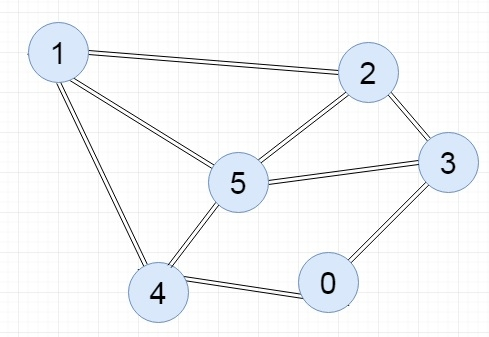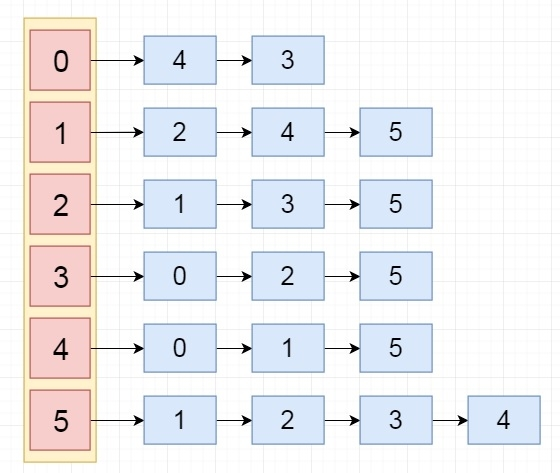# C++ Program to Implement Adjacency List

The adjacency list representation of a graph is linked list representation. In this representation we have an array of lists The array size is V. Here V is the number of vertices. In other words, we can say that we have an array to store V number of different lists. If a list header is vertex u, then it signifies that it will hold all of the adjacent vertices of u.

### The complexity of Adjacency List representation

• This representation takes O(V+2E) for undirected graph, and O(V+E) for directed graph. If the number of edges are increased, then the required space will also be increased.

Input:Output:## Algorithm

Input: The u and v of an edge {u,v}, and the adjacency list

Output: Adjacency List of the graph G

Begin
Append v into the list at index u
Append u into the list at index v
End

## Example Code

#include<iostream>
#include<list>
#include<iterator>
using namespace std;
for(int i = 0; i<v; i++) {
cout << i << "--->";
list<int> :: iterator it;
cout << *it << " ";
}
cout << endl;
}
}
void add_edge(list<int> adj_list[], int u, int v) {    //add v into the list u, and u into list v
}
main(int argc, char* argv[]) {
int v = 6;    //there are 6 vertices in the graph
//create an array of lists whose size is 6
}
0--->4 3
5--->1 2 3 4